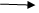# How to Implement LINQ Queries in Collection Data

Blog Author

Tekslate

Published Date

21st September, 2018

Ratings

Views

835

## Implementing LINQ  Queries on Collection Data

Create  a new Console Application   (CA Sample 2)  àAdd a new class (Name) à Employee Write the following code using Auto implemented properties.

```Name Space CA Sample 2
{
Class Employee
{
Public Int PEMP ID {set; get;}
Public String PE name  {set; get ;}
Public String Designation  {set; get ;}
Public Date time PDOJ  {set; get ;}
Public Double Salary  {set; get ;}
Public Int p Dept no {set; get ;}
}
}

```
`Learn the core features of ASP.NET Training and become master with our expertise tutorials.`

### Create a new class in this Application with the name Examples:-

Write the following code

```Namespace CA Sample 2
{
Class Example2
{
Static void Main ()
{
List <Employee> emp list = new list <Employee> ()
{
New  employee  (P emp id = 101, P Ename = “naveen”, Pdesigen = “pl”, p Doj = new date (2012,03,02),p salary = 1200, pdeptno =10);
New  employee  (P emp id = 102, P Ename = “raj”, Pdesigen = “tl”, p Doj = new date (2012,04,01),p salary = 1300, pdeptno =20);
New  employee  (P emp id = 103, P Ename = “sai”, Pdesigen = “ds”, p Doj = new date (2012,05,03),p salary = 1300, pdeptno =10);
};
Var R = from X in Emp list where x.pdept no = 10  Select x;
Console. Write line (“Dept no 10 employee data is :-”);
Console. Write line (“Empid/t, ename/t, designation/t, doj/t salary/t……………”);
Foreach (var I in R)
{
Console. Write line (i.pempid +’/t’  + i. pename +’/t’ +i.pdesig +’/t’+ i.p doj +’/t’+ i.p salary);
}
}
}
}```

### Output :-

Emp id                       E name                   designation                  Doj         salary 101                                  -                               -                                   -            1200 103                                  -                                -                                  -              1400If we want to only required fields we use query like.Var R = from  x in emplist where x. p dept no = 10 select  x.ename, x. designation, x.esalary;

For indepth understanding click on